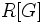# Centralizer ring

(diff) ← Older revision | Latest revision (diff) | Newer revision → (diff)
Given a group$G$, a subgroup$B$, and a ring$R$, the centralizer ring of$G$ with respect to the subgroup$B$ and over the ring$R$ is defined in any of the following equivalent ways:
• It is the endomorphism ring of the$R[G]$-module$R[G/B \times G/B]$ where the module action is defined by coordinate-wise left multiplication by$G$
• It is the endomorphism ring of the$R[B]$-module$R[G/B]$ where$B$ acts on the coset space by left multiplication.
• It is the endomorphism ring of the$R$-module over the double coset space of$B$ in$G$.
In the particular case where$G$ is an algebraic group and$B$ is a Borel subgroup, the corresponding centralizer ring is called the Hecke algebra.